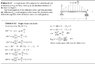# Deflection by integration of load equation

• Freyster98

## Homework Statement

Determine the equation for the deflection curve for the cantilever supported at A with a load given by: q=q0*sin($$\pi$$x/L).

## The Attempt at a Solution

I think this is pretty straightforward, but want to be sure. I did a similar problem with a simply supported beam with the same load equation, shown in the attached diagram. Am I safe to assume that the ONLY difference in this problem with a cantilever is the boundary conditions used to solve for C1, C2, etc?

The boundary conditions would be:
at x=0,y=0...and at x=0,moment=0.
right?

#### Attachments

•46.5 KB · Views: 612